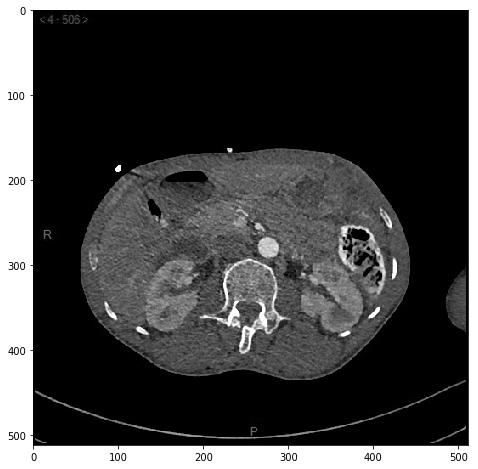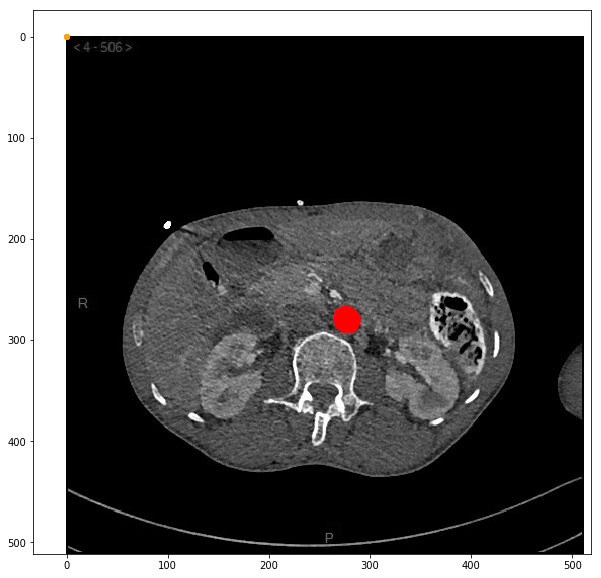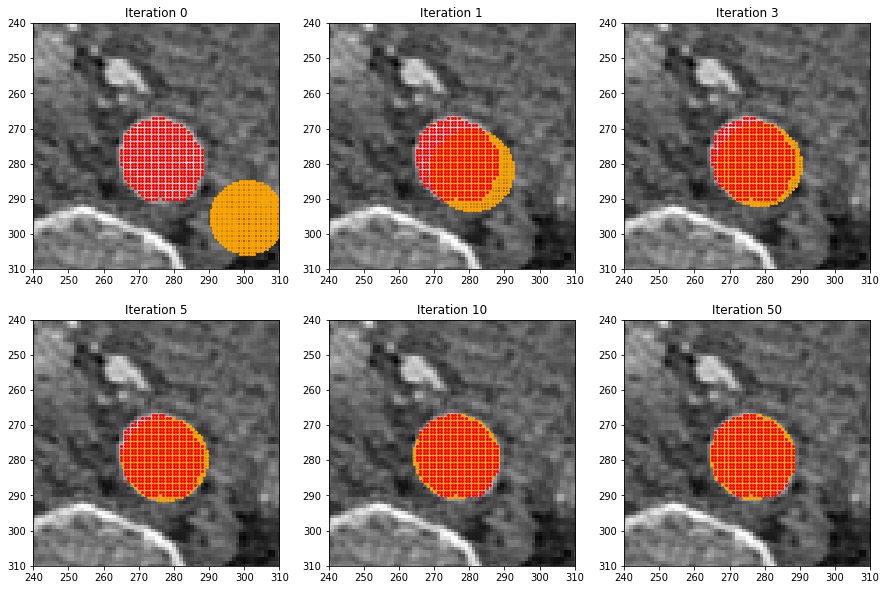# Part 5: Shape registration with gradient descent¶

In the previous notebook, we've seen that geometric analysis is best performed on point clouds instead of bitmaps. We're now going to present an iterative method that can be used to fit arbitrary models to datasets: gradient descent.

As usual, we first need to re-import standard modules, re-defining our display and extract_points routines:

In :
%matplotlib inline
import center_images             # Center our images
import matplotlib.pyplot as plt  # Display library
import numpy as np               # Numerical computations

def display(im):  # Define a new Python routine
"""
Displays an image using the methods of the 'matplotlib' library.
"""
plt.figure(figsize=(8,8))                     # Square blackboard
plt.imshow( im, cmap="gray", vmin=0, vmax=1)  # Display 'im' using a gray colormap,
#         from 0 (black) to 1 (white)

"""
Turns a binary mask (bitmap) into a list of point coordinates (an (N,2) array).
"""
return np.vstack(mask.nonzero()).astype(float).T[:,::-1]  # Dark magic with NumPy...


Once again, we work on a simple axial slice:

In :
I = imread("data/aortes/1.jpg", as_gray=True)  # Import as a grayscale array
I = I / 255                   # Normalize intensities in the [0,1] range
display(I)                    # Let's display our slice:The template x_0 and target y are defined as in Part 4:

In :
# Template x_0 = the unit disk -----------------------------------------------

Y, X = np.mgrid[:31, :31]   # Generates horizontal and vertical gradients of size 31x31
distance = (X - 15) ** 2 + (Y - 15) ** 2  # Squared distance to the point (15,15)
disk = distance <= 15.5**2  # Disk = mask of points which are close to (15,15)
x_0 = extract_points(disk)  # Extract the points' coordinates
x_0 = (x_0 - 15)/30   # Center (mean = 0) and normalize (diameter = 1) the point cloud

# Target y = the segmented aorta ---------------------------------------------

from scipy.ndimage.morphology import binary_erosion, binary_dilation

mask   = (I > .6) & (I < .9)                   # Threshold the image values
core   = binary_erosion( mask, iterations=5)   # Remove the thin components
region = binary_dilation(core, iterations=10)  # Core growth -> trust region
aorta  = (I > .6) & region                     # Intersection between "image is
#  not too dark" and the trust region
y = extract_points(aorta)                      # Binary mask -> point cloud

# Display everyone -----------------------------------------------------------
plt.figure(figsize=(10,10))                      # Create a square blackboard
plt.scatter(x_0[:,0], x_0[:,1], s=9, c="orange") # Display the model 'x_0' in orange
plt.scatter(  y[:,0],   y[:,1], s=9, c="red")    # Display the target 'y' in red
plt.imshow(I, cmap="gray") ; plt.axis("equal");  # Display the slice in the background### Minimizing the Energy Distance through gradient descent¶

Question is: How are we going to fit a model x = move(x_0, a,o, w,h) to the segmented point cloud y?

In the previous notebook, we've seen that the mean and standard deviations of y could be used as reasonable estimates for the parameters a, o, w and h... But this approach can hardly be generalized to real-life settings, where the deformation to consider is more complex than a simple translation + stretching.

In most applications, engineers can at best make a wish: the parameters should be those that minimize the (Energy) distance $\text{D}$ between the model x and the target y. In mathematical jargon:

\begin{align} a^\star, o^\star, w^\star, h^\star ~~ =~~ \arg\min_{a,o,w,h} ~\underbrace{\text{D}(\, \text{move}(x_0,a,o,w,h)~;~y\,)}_{\text{Loss}(a,o,w,h)}, \end{align}

as we try to minimize our custom $\text{Loss}$ function with respect to the tunable parameters a, o, w and h.

Gradient descent. Optimization problems, where one strives to minimize a cost under constraints, have been at the heart of applied mathematics for the last 200 years. As you'd guess, researchers have come up with super-efficient and generic methods, typically bundled in the scipy.optimize and torch.optim packages to be used as black boxes. But under the hood, most of these routines rely on a simple, intuitive idea: gradient descent.

If $f : x\in\mathbb{R}\mapsto f(x)\in\mathbb{R}$ is a differentiable function, we know that $f$ behaves locally like its tangent: for variations $h$ that are small enough,

\begin{align} f(x+h)~\simeq~ f(x)~+~ f'(x)\cdot h. \end{align}

Consequently, the derivative $f'(x)$ at a point $x$ is:

• positive if $f$ is locally increasing,
• null if $x$ is a local extremum,
• negative if $f$ is locally decreasing.

\begin{align} x^{(k+1)}~=~x^{(k)}~-~\text{lr}\cdot f'(x^{(k)}), \end{align}

with an arbitrary starting estimate $x^{(0)}$ and a small enough learning rate $\text{lr}>0$, the sequence $x^{(0)}$,..., $x^{(k)}$,... should produce decreasing values $f(x^{(k)})$ of the cost function: at every iteration, we make a small step against the derivative.

Eventually, this process converges towards a critical point $x^\star$ of the function $f$, such that $f'(x^\star) =0$: If the function $f$ to minimize is well-behaved (convex), this is our optimum. Problem solved!

With four parameters. In our case, the $\text{Loss}$ function to minimize doesn't have one, but four parameters. No worries: assuming that we can compute the gradient vector of partial derivatives

\begin{align} \frac{\partial\text{Loss}}{\partial a}, ~~ \frac{\partial\text{Loss}}{\partial o}, ~~ \frac{\partial\text{Loss}}{\partial w} ~~ \text{and}~~ \frac{\partial\text{Loss}}{\partial h}, ~ \end{align}

which generalizes the derivative $f'$, iterating parallel updates

\begin{align} a^{(k+1)}~&=~a^{(k)}~-~\text{lr}\cdot \frac{\partial\text{Loss}}{\partial a}(a^{(k)},o^{(k)},w^{(k)},h^{(k)}), \\ o^{(k+1)}~&=~o^{(k)}~-~\text{lr}\cdot \frac{\partial\text{Loss}}{\partial o}(a^{(k)},o^{(k)},w^{(k)},h^{(k)}), \\ w^{(k+1)}~&=~w^{(k)}~-~\text{lr}\cdot \frac{\partial\text{Loss}}{\partial w}(a^{(k)},o^{(k)},w^{(k)},h^{(k)}), \\ h^{(k+1)}~&=~h^{(k)}~-~\text{lr}\cdot \frac{\partial\text{Loss}}{\partial h}(a^{(k)},o^{(k)},w^{(k)},h^{(k)}) \end{align}

will do the trick!

Chain-rule and backpropagation. Needless to say, this method relies on a fast and efficient computation of partial derivatives... Which I cannot really explain here: derivations are a bit tedious, and probably too technical for physicians.

If you're still curious anyway, you may have a look at the session 10 of my data science workshop notes, which details the theory behind these lines of code. Otherwise, you can simply trust me on this: the routine loss_and_gradient defined below is correct!

In :
def move(x_0, a,o, w,h):
"""
Stretches and translates a given point cloud 'x_0'.
The input parameters are the 'abscisse', the 'ordonnée',
the 'width' and the 'height' of the final destination.
"""
return x_0 * [w,h] + [a,o]

def grad_move(x_0, a,o, w,h, g_x) :
"""
Takes as input a gradient 'g_x' with respect to the output of
'move(x_0, a,o, w,h)', and returns four derivatives with
respect to 'a', 'o', 'w' and 'h'.
"""
g_a,g_o = g_x.sum(0)          # Abscisse and Ordonnée : use the formulas above
g_w,g_h = (x_0 * g_x).sum(0)  # Width and Height      : use the formulas above

return g_a,g_o, g_w,g_h       # This routine returns four numbers

In :
from scipy.spatial import distance_matrix

def D(x, y) :
"""
Computes the 'Energy Distance' between two point clouds.
"""
N, M = len(x), len(y)
XY = distance_matrix(x,y).sum() / (N*M)  # = (1/NM) * ∑_ij |x_i-y_j|
XX = distance_matrix(x,x).sum() / (N*N)  # = (1/NN) * ∑_ij |x_i-x_j|
YY = distance_matrix(y,y).sum() / (M*M)  # = (1/MM) * ∑_ij |y_i-y_j|
return XY - (XX+YY)/2                    # See the equation above

"""
Computes the gradient with respect to 'x' of the Energy Distance.
"""
N, M = len(x), len(y)
XY = distance_matrix(x,y)[:,:,None]  # (N,M) matrix of pairwise distances |x_i-y_j|
XX = distance_matrix(x,x)[:,:,None]  # (N,N) matrix of pairwise distances |x_i-x_j|
XmY = x[:,None,:] - y[None,:,:]      # (N,M,2) matrix of coordinates, (x_i-y_j)[k]
XmX = x[:,None,:] - x[None,:,:]      # (N,N,2) matrix of coordinates, (x_i-x_j)[k]
g_xy = ( XmY/(XY+.00001) ).sum(1).reshape(N,2) / (N*M)  # Sum wrt. "j" in [1,M]
g_xx = ( XmX/(XX+.00001) ).sum(1).reshape(N,2) / (N*N)  # Sum wrt. "j" in [1,N]
g_x = g_xy - g_xx     #  = the formula detailed in the equation above
return g_x    # g_x is an (N,2) array, that should be understood as a vector field

In :
def loss_and_gradient(x_0, y, a,o, w,h) :
"""
Computes the Loss to minimize and its partial derivatives with respect to the
deformation's parameters.

This routines takes as input a template 'x_0', a target point cloud 'y',
an abcissa 'a', an ordinate 'o', a width 'w' and a height 'h'.
"""
# Forward pass : we define a loss with respect to some parameters -----------------
x = move(x_0, a,o, w,h)  # Our model, an ellipsoid of center (a,o) and shape (w,h)
loss = D(x,y)  # "Energy Distance" between the model 'x' and the segmented aorta 'y'

# Backward pass : we compute our partial derivatives with respect to the parameters
# N.B.: This code is in perfect "symmetry" with the forward pass above, and can be
#       generated automatically (look up for the "backpropagation" algorithm,
#       implemented in the PyTorch and TensorFlow libraries).
g_x = grad_D(x,y)  # Partial derivative wrt. the positions of the points 'x_i'
g_a,g_o, g_w,g_h = grad_move(x_0, a,o, w,h, g_x)  # Partial derivative wrt.
# 'a', 'o', 'w' and 'h'
# This information will be used to perform "gradient descent":
return loss, g_a,g_o, g_w,g_h


The routine above allows us to compute $\text{Loss}$ and its partial derivatives for arbitrary values of the parameters a, o, w and h. Starting from an arbirary estimate, we can now use our iterative algorithm to register the model ellipse x onto the aorta y:

In :
# Initialize the model's parameters with reasonable first guesses:
a,o = y.mean(0) + [45,30]  # As seen in Part 4, the best guess would be "y.mean(0)" and
w,h = 3*y.std(0)           # "4*y.std(0)", but our registration pipeline is robust :-)

lr = 25.  # Learning rate, which controls the step size in our descent

plt.figure(figsize=(15,10))  # Rectangular blackboard
save_its = [0,1,3,5,10,50]   # Iterations that we want to display

for i in range(51) :         # Loop on i = [0, 1, 2, ..., 50]

# Compute the "Energy Distance" and its partial derivatives:
loss, g_a,g_o, g_w,g_h = loss_and_gradient(x_0, y, a,o, w,h)
a = a - lr*g_a ; o = o - lr*g_o  # Update the ellipsoid's position
w = w - lr*g_w ; h = h - lr*g_h  # Update the ellipsoid's shape

# Fancy display ---------------------------------------
print("{:.4f}".format(loss), end=", ")  # Monitor the descent
if i % 10 == 0 : print("")              # Break line every ten iterations

if i in save_its :
plt.subplot(2,3,save_its.index(i)+1)  # Display iterations in a 2x3 waffle plot
x = move(x_0, a,o, w,h)               # Re-compute the model point cloud...
plt.scatter(x[:,0], x[:,1], s=4, c="orange")  # and display it in orange.
plt.scatter(y[:,0], y[:,1], s=4, c="red")     # The target is in red...
plt.imshow(I, cmap="gray")            # and the raw data, in the background.
plt.axis([240,310,240,310])           # Crop the view around the aorta
plt.title("Iteration {}".format(i))   # Set the subfigure's title
plt.gca().invert_yaxis()              # The y-axis points towards the bottom

print("Done.")
print("width = {:0.2f} pixels, height = {:0.2f} pixels".format(w,h))

44.9195,
20.1068, 0.9545, 0.4262, 0.2104, 0.1092, 0.0586, 0.0326, 0.0189, 0.0116, 0.0077,
0.0056, 0.0045, 0.0038, 0.0035, 0.0033, 0.0032, 0.0032, 0.0031, 0.0031, 0.0031,
0.0031, 0.0031, 0.0031, 0.0031, 0.0031, 0.0030, 0.0030, 0.0030, 0.0030, 0.0030,
0.0030, 0.0030, 0.0030, 0.0030, 0.0030, 0.0030, 0.0030, 0.0030, 0.0030, 0.0030,
0.0030, 0.0030, 0.0030, 0.0030, 0.0030, 0.0030, 0.0030, 0.0030, 0.0030, 0.0030,
Done.
width = 23.44 pixels, height = 23.38 pixelsIt works! Even for sub-optimal initializations, the gradient of our $\text{Loss}$ function acts as a spring, which drives the model x (in orange) towards the target y (in red).

To remember: Optimization methods allow us to "fit" any set of parameters to a dataset by flowing along the gradient of a $\text{Loss}$ (or "Energy") function. These updates simulate what happens when a ball rolls along a slope, until reaching a basin centered around the target.

In this notebook, we used this algorithm to fit an ellipse onto an aorta. But in the next sessions of the Masterclass, you will see that this simple algorithm is also the standard method to fit the weights of a Convolutional "Neural Network" onto a specific database. When researchers speak about "machine learning" and "training", you should always remember that they refer to this simplistic, physics-based algorithm that generalizes linear regression to arbitrary $\text{Loss}$ functions.

Not one bit of "reflection" or "thought" is involved!